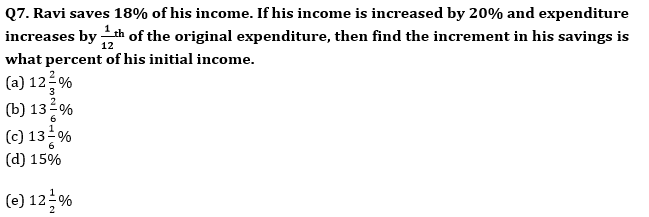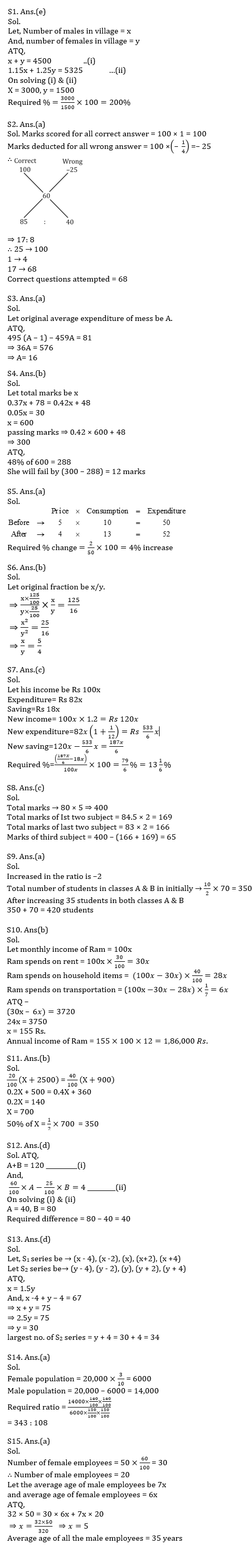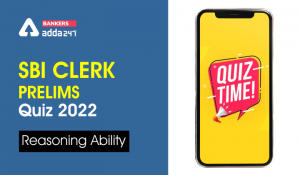Latest Banking jobs   »   Quantitative Aptitude Quiz For SBI PO,...

# Quantitative Aptitude Quiz For SBI PO, Clerk Prelims 2021- 17th June

Q1. The population of a village is 4500. If number of males increases by 15% and number of females increases by 25% then the population of village become 5325. Number of males is what percent of the Number of females in the village.
(a) 100%
(b) 125%
(c) 150%
(d) 175%
(e) 200%

Q2. A student get +1 mark four each correct answer and (–1/4) mark for each wrong answer. If there were total 100 questions in a paper and a student scored 60 marks by attempting all question. Find the number of correct question attempted by him?
(a) 68
(b) 56
(c) 74
(d) 70
(e) 66

Q3. In a hostel consisting of 459 students, if number of students increases by 36, the expense of mess per day increased by Rs. 81 while reducing the average expense per head by Rs. 1, Find the original expenditure of mess ?
(a) 16
(b) 15.5
(c) 15
(d) 16.5
(e) 18

Q4. Neha scored 37% marks and failed by 78 marks. If she scored 42% marks, she fails by 48 marks. Find by how much marks would she fail or pass if she scored 48% marks?
(a) 16 marks,fail
(b) 12 marks,fail
(c) 10 marks,pass
(d) 18 marks,pass
(e) 25 marks, fail

Q5. The price of sugar decreases by 20% and consumption of a family increased by 30%. Find the % change in the expenditure of family?
(a) 4% increase
(b) 2.5% increase
(c) 4% decrease
(d) 2.5% decrease
(e) 5% increase

Q6. The numerator of a fraction is increased by 25% and denominator decreased by 75% . The product of new fraction formed and original fraction is 125/16. Find original fraction?
(a) 5 : 3
(b) 5 : 4
(c) 4 : 3
(d) 3 : 5
(e) 4 :7

Q8. Average marks of a student in five subjects is 80. If the average marks of first two subject is 84.5 and the average marks of last two subject is 83. Find the marks of third subject ?
(a) 51
(b) 53
(c) 65
(d) 63
(e) 60Q9. The number of students in three classes A, B and C in the ratio of 3 : 7 : 5. If 70 students are increased in each classes, the ratio changes to 5 : 9 : 7. Then find the total number of students in class A and B if 35 students increased in both the classes.
(a) 420
(b) 400
(c) 350
(d) 370
(e) 380

Q10. Ram spends 30% of his monthly income on rent, 40% of remaining on household items and 1/7 th of remaining on transportation. If difference between amount spent on rent & on transportation by Ram is Rs. 3720, then find annually income of Ram?
(a) 1,89,000
(b) 1,86,000
(c) 1,76,000
(d) 1,48,000
(e) 1,88,000

Q11. 20% of (X + 2500) is equal to the 40% of (X + 900). Then find 50% of X ?
(a) 250
(b) 350
(c) None of these
(d) 450
(e) 150

Q12. Average of two number A and B is 60. If 60% of A is four more than 25% of B then find the difference between A and B?
(a) 10
(b) 20
(c) 30
(d) 40
(e) 50

Q13. ‘S1’ is a series of five consecutive odd number while ‘S2’ is a series of five consecutive even numbers. Average of S1 series is 50% more than average of S2 series and sum of smallest numbers of S1 and S2 series is 67, then find the largest number of S2 series
(a) 49
(b) 41
(c) 36
(d) 34
(e) 32

Q14. The present population of a city is 20,000. The ratio of female to male is given as 3 : 7. If population of female increases by 20% per year and population of male increases by 40% per year then find the ratio of male to female after two years in the city ?
(a) 343 : 108
(b) 102 : 343
(c) 341 : 108
(d) 108 : 343
(e) 343 : 102

Q15. Average age of all the employees in a company is 32 years. 60% of the employees in the company are female and the ratio of the average age of all female to average age of all male employees is 6 : 7. Find the average age of all the male employees if there are total 50 employees in the company.
(a) 35 years
(b) 42 years
(c) 28 years
(d) 49 years
(e) 30 years

Practice More Questions of Quantitative Aptitude for Competitive Exams:

###### SBI Clerk Study Plan 2021

Solutions#### Congratulations!

Incorrect details? Fill the form again here

•Quantitative Aptitude Quiz For SBI Clerk...
•English Quizzes For SBI Clerk Prelims 20...
•Reasoning Ability Quiz For SBI Clerk Pre...
•Quantitative Aptitude Quiz For SBI Clerk...
•Quantitative Aptitude Quiz For SBI Clerk...
•Reasoning Ability Quiz For SBI Clerk Pre...